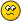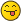# Tetration Forum

Full Version: The Different Fixed Points of Exponentials
You're currently viewing a stripped down version of our content. View the full version with proper formatting.
Pages: 1 2 3
Is there a pattern to the positions of different fixed points of exponentials? Like is there a pattern to the positions of different fixed points of ? What about for the fixed points of ? How are they arranged in the complex plane? Are there more fixed points of exponentials in the quaternions? Or what about in the Octonions?
Compare the norm of exp iterated n times with the identity
There does seem to be a pattern to the positions, in the complex plane of the different fixed points of exp(z).
There used to be a graph of , from -32 to 32 in both directions attached to this post, but I removed it try to replace it with a better one.
I apologize for my fractal "n00bness"... but is there available a diagram of the complex plane showing in addition to the fixed points also the shapes and positions of their respective basin of attraction? I'm interested in visualizing the sets $$A_p=\{z\in\mathbb C\, :\, \lim_{n\to \infty} \exp_b ^n(z)=p\}$$.

What is the knowledge about the set $$A=\bigcup_{p\in {\rm fix}(\exp_b)}A_p$$ and the set $$\mathbb C\setminus A$$. Are they open? Closed? Connected by arcs? Are they punctured/have holes?
(07/07/2022, 08:29 PM)MphLee Wrote: [ -> ]I apologize for my fractal "n00bness"... but is there available a diagram of the complex plane showing in addition to the fixed points also the shapes and positions of their respective basin of attraction? I'm interested in visualizing the sets $$A_p=\{z\in\mathbb C\, :\, \lim_{n\to \infty} \exp_b ^n(z)=p\}$$.

What is the knowledge about the set $$A=\bigcup_{p\in {\rm fix}(\exp_b)}A_p$$ and the set $$\mathbb C\setminus A$$. Are they open? Closed? Connected by arcs? Are they punctured/have holes?

Hey, Mphlee

I believe your question isn't the question you intended to ask. So first I'll answer the question you did ask, and then extrapolate what I think you meant to ask.

First of all, the only attracting fixed points are in the Shell-Thron region. So you are asking for what values z are in the basin of the fixed points.

The fixed points come in a set that looks like $$|\log(p)| < 1$$, and then $$b = e^{\log(p)/p}$$. The basin of these fixed points are very sensitive, and nonsensical. There is not much literature that I've found describing them.

An important fact, is when $$b \in (1,\eta)$$, that $$\Re(z) < 0$$ is in the basin. As you move in the complex plane, this half plane deforms, I believe there's always a half plane within $$A_p$$ for all such $$p$$--not sure how to describe it. But don't quote me on that, I'm not certain.

Then when you ask for your set $$A$$, it can be written more clearly as:

$A = \{z \in \mathbb{C}\,|\, \exists p\, \text{such that}\,|\log(p)| < 1,\,\lim_{n\to\infty}\exp_{p^{1/p}}^{\circ n}(z) = p\}\\$

This set isn't all that interesting, at least I don't think so. It is definitely open, just by looking at this definition. It is probably simply connected, as it's the union of simply connected domains that are deformations of each other. This means there shouldn't be any holes.

The more interesting chaos, and the much crazier idea, would be to invert your definition of $$A_p$$. Lets call it $$B_p$$:

$B_p = \{z \in \mathbb{C}\,|\, \lim_{n\to\infty} \log^{\circ n}_b(z) = p\}\\$

This would produce MANY MANY more fixed points. This would be a much more interesting beast, as it'd deal with fixed point pairs of the exponential, and the ridiculous amount of fixedpoints that the exponential has (depending on how you choose the signature of each log in the iteration). The thing is, pretty much all the fixed points are repelling. The only attracting ones are in the shell-thron region. This means, the fixed points are attractive in $$\log$$ though. And choosing each branch of $$\log$$ as you iterate, produces different fixed points. And these are a much more interesting version of your set. It's kinda like the compliment you brought up. Instead of defining $$A_p$$ through attracting fixed points of $$\exp_b$$, which are well understood. Look at $$B_p$$ defined the exact same manner off of repelling fixed points.

Also, to Catullus' question, there is a pattern to the fixed points.

In Devaney's book "An intro to chaotic dynamical systems" He has a large section on iterating:

$\lambda e^z\,\,\text{for}\,\,0 < \lambda\\$

He describes the structure of the Julia set/fatou sets using symbolic dynamics. It's far too involved to describe here, but it's a standard modeling technique in dynamics. Devaney describes how different parts of the complex plane maps to other parts under iterations, and on intersections of these domains we have the fixedpoints/periodic points. It's absolutely fascinating. I'm not that well versed in it, but to start you off, search for the symbolic dynamics of the exponential functions. Or pick up Devaney's bookI totally forgot Devaney's book! It's amazing. It was on my todo list since ages. Now I'm eating it like bread... many things are super clear now...
I think that after it I can begin something more juicy and than straight to Milnor... maybe before september If I manage to read carefully I can parse 60% of this forum's posts.

I'll be back on this post when I've finished Devaney... I hope I'll be able to find time for some exercises tooOff topic, reading Devaney on symbolic dynamics I just remember that Lawere proposed an algebraic definition of chaos. Let $${\bf X}=(X,f)$$ be a dynamical system. Let $$A^\mathbb N=(A^{\mathbb N},\sigma)$$ be the shift space over the alphabet $$A$$. Given an observable $$\phi:X\to A$$ that assign to every state a measurement, there always exists a canonical dynamical systems map $${\bar \phi}:{\bf X}\to A^\mathbb N$$ assigns what Devaney calls "itinerary" (of observations). $x \mapsto (\phi(x),\phi(f^1(x)),\phi(f^2(x)),..., \phi(f^n(x)),...)$

The observable $$\phi$$ is said chaotic if $$\bar \phi$$ is surjective. It means that the observable is so weak that we can observe every possible behavior... we call this chaotic behavior.

The reason I asked the question is because I wanted to have a mental picture of the dynamics of exponentiation in term of graphs/phase protrait.
Oh yes!

Thank you Mphlee for reading Devaney first. Oddly enough it was a harder book for me compared to Milnor, but that's because I'm analytic calculus (trigonometric reasoning). Devaney was hard for me because of the algebraic stuff; kinda made my head spin. Makes sense that Devaney would be easier for you, lmao.

Also, do not be intimidated by Milnor. He gives great break downs of everything in plain english. If you miss some of the proofs, that's okay. You always know that these proofs are 100%. And Milnor is literally like the Shakespeare of mathematics. Guy writes math so clearly and explanatory. You can skip parts if you don't get it, not a problem with Milnor. He reads like Hamlet, where really all it is is a bunch of soliloquy, and they're all stand alone.

There's the old joke that John A. Milnor Complex Dynamics is the modern bible. And anyone studying stuff like this needs to read it. Who cares if you don't actually get how he got that bound!! Just watch how he uses it. And the logical structure. The shape of the arguments. So you didn't get why this limit argument works--you can trust that it's true because it's Milnor. But watch how he uses it...

Devaney is great, but he's no Milnor. Milnor fucking fucked shit up with that book, Mphlee. We can all hope to be so successfulI removed the attachment from #3, because it had some issues.
I might add a better attachment to #3.
Hmm, I'm not going into this matter deeply at the moment.
I had looked at the question of location of fixpoints and a possible interpolation-line once in 2008 but had not developed this much. At least there is a picture showing the attempt:
[attachment=1771]
Pages: 1 2 3